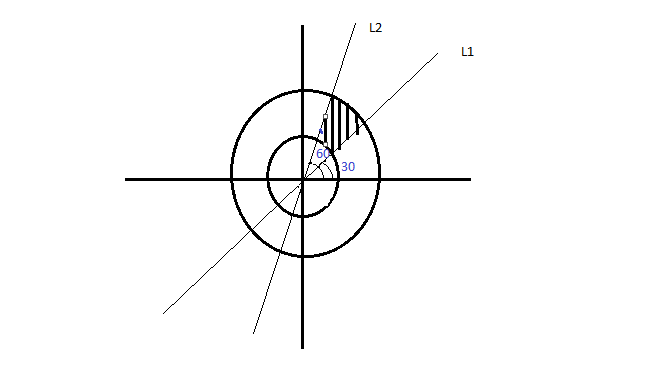QuestionAnswers

# The area bounded by the circles ${x^2} + {y^2} = 1$ and ${x^2} + {y^2} = 4$ , and the pair of lines $\sqrt 3 ({x^2} + {y^2}) = 4xy$ in the first quadrant is equal to:$(1){\text{ }}\dfrac{\pi }{2} \\ (2){\text{ }}5\dfrac{\pi }{2} \\ (3){\text{ }}3\pi \\ (4){\text{ }}\dfrac{\pi }{4} \\$Hint: Here we have given two equations of circle and a pair of straight lines is given so first of all we have to find individual equations of straight line and then draw a diagram of all the curves given and then shade the region in first quadrant which is formed by all four equations. And now we have to just find the area of that shaded diagram.

Given- Circle 1: ${x^2} + {y^2} = 1$ and Circle 2: ${x^2} + {y^2} = 4$
Pair of lines: $\sqrt 3 ({x^2} + {y^2}) = 4xy$
Find the individual equations of the straight lines. We have
$\sqrt 3 {x^2} - 4xy + \sqrt 3 {y^2} = 0$
Factorizing,$\sqrt 3 {x^2} - 3xy - xy + \sqrt 3 {y^2} = 0$
Simplifying,$(x - \sqrt 3 y)(\sqrt 3 x - y)$
Line 1: $x - \sqrt 3 y = 0$ Line 2: $\sqrt 3 x - y = 0$We need to find the shaded area. For this, we will use the sector formula.
When area of only a sector of a circle covering $\theta$ angle is to be found:
$\dfrac{\theta }{{2\pi }} \times \pi {r^2}$
Here, since we have to find the area of the region between two circles:$\dfrac{\theta }{{2\pi }} \times \pi [r_2^2 - r_1^2]$
The angle $\theta$ here is (60 - 30 = 30) i.e. $\dfrac{\pi }{6}$
${r_2} = 2{\text{ , }}{{\text{r}}_1} = 1$
On solving, we get
Area = $\dfrac{\pi }{4}$ .

So, the correct answer is “Option 4”.

Note: Whenever we get this type of question the key concept of solving is we should have knowledge on how to find individual equations of straight line when the equation of pair of straight lines is given. The formula of area of sector should be remembered. Area of sector = $\dfrac{\theta }{{2\pi }} \times \pi {r^2}$.
Area of a Sector of a Circle FormulaCBSE Class 10 Maths Chapter 10 - Circles FormulaCBSE Class 10 Maths Chapter 13 - Surface Areas and Volumes FormulaChanging the Period of a PendulumThe Making of a ScientistArea of TrapeziumArea of a Rhombus FormulaThe Idea of TimeWhat are the Functions of the Human Skeletal System?Area of Hollow CylinderImportant Questions for CBSE Class 10 Maths Chapter 10 - CirclesImportant Questions for CBSE Class 10 Maths Chapter 12 - Areas Related to CirclesImportant Questions for CBSE Class 6 English A Pact with The Sun Chapter 6 - The Monkey and the CrocodileImportant Questions for CBSE Class 11 English Snapshots Chapter 1 - The Summer of the Beautiful White HorseImportant Questions for CBSE Class 9 Maths Chapter 10 - CirclesImportant Questions for CBSE Class 7 English Honeycomb Chapter 10 - The Story of CricketImportant Questions for CBSE Class 8 Science Chapter 10 - Reaching The Age of AdolescenceImportant Questions for CBSE Class 6 Social Science The Earth Our Habitat Chapter 1 - The Earth In The Solar SystemCBSE Class 8 Science Reaching The Age of Adolescence WorksheetsImportant Questions for CBSE Class 6 English A Pact with The Sun Chapter 1 - A Tale of Two BirdsCBSE Class 10 Maths Question Paper 2017CBSE Class 10 Hindi A Question Paper 2020Hindi A Class 10 CBSE Question Paper 2009Hindi A Class 10 CBSE Question Paper 2015CBSE Class 10 Maths Question Paper 2020Hindi A Class 10 CBSE Question Paper 2008Hindi A Class 10 CBSE Question Paper 2014Hindi A Class 10 CBSE Question Paper 2007Hindi A Class 10 CBSE Question Paper 2013Hindi A Class 10 CBSE Question Paper 2016NCERT Solutions for Class 10 Maths Chapter 12 Areas Related to CirclesRD Sharma Solutions for Class 10 Maths Chapter 15 - Areas Related to CirclesNCERT Solutions for Class 10 Maths Chapter 10 CirclesRD Sharma Solutions for Class 10 Maths Chapter 10 - CirclesNCERT Solutions for Class 9 Maths Chapter 10 CirclesRS Aggarwal Class 10 Solutions - CirclesRS Aggarwal Class 12 Solutions Chapter-17 Area of Bounded RegionsNCERT Solutions for Class 10 Social Science India and the Contemporary World - II Chapter 1 - The Rise of Nationalism in EuropeNCERT Solutions for Class 10 Maths Chapter 13 Surface Areas and VolumesNCERT Solutions for Class 10 Social Science India and the Contemporary World - II Chapter 4 - The Making of a Global World